# TI-30X Pro MultiView™ Scientific Calculator

Advanced four-line scientific calculator, with higher-level maths and science functionality. Ideal for students in GCSE, A/AS and Further Maths levels. TI-30X Pro meets the new requirements in the new A-level curriculum. Even fit for Further Maths level.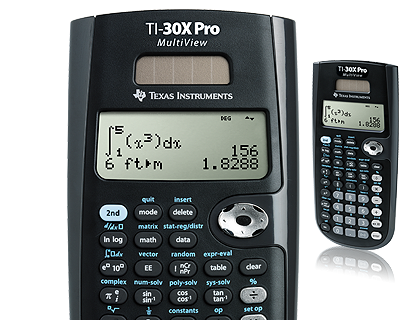• Four-line display
• MultiView™ display shows multiple calculations at the same time on screen
• Meets GCSE requirements: fraction keys and prime factorizing; simple power and root facilities; exact surd arithmetic.
• Meets all GCE-A/AS level DfE specifications: iterative function, compute summary statistics and access probabilities from standard statistical distributions; matrix calculations and inverses up to 3x3; all the usual regression and correlation functions; calculation of probabilities for a binomial distribution and normal distribution.
• AS Further Maths functionality: calculation of Poisson distribution.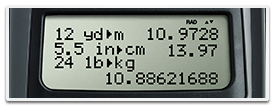## View multiple calculations at the same time

Compare results and explore patterns on-screen with the MultiView™ display, which supports up to four lines.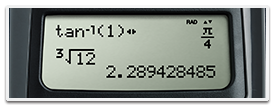## See math exactly as it appears in textbooks

See math expressions, symbols and stacked fractions exactly the way they appear in textbooks — no need to adapt to a technical syntax; also provides quick access to frequently used functions.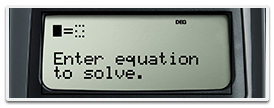## Built-in solvers

Intuitive prompts allow one to easily solve numeric equation, polynomial and system of linear equations.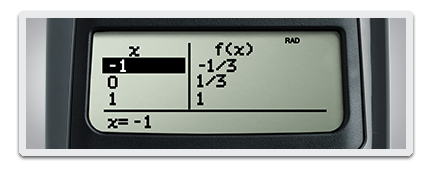## Explore (x,y) table of values

Students can easily explore an (x,y) table of values for a given function, automatically or by entering specific x values..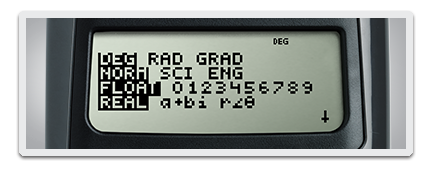## Easy-to-use mode menu

The menu is structured for easy, intuitive access to commands; select degrees/radians, floating/fix, Classic/MathPrint™ or number format.## Specifications#### Built-in functionality

• Review and edit previous entries via a scrollable home screen
• Paste inputs or outputs into new calculations
• MathPrint™ entry and output mode for viewing calculations in maths notation, including answers in terms of pi, square roots and fraction
• Three solvers – numeric equation, polynomial and system of linear equations
• Numeric derivative and integral for real functions
• Vectors and Matrices
• Symbolic notation of π
• Toggle key to change the form of answers between exact and decimal approximation
• Stacked Fractions and fraction functions
• Fraction/decimal/percent conversions
• Change between improper fractions and mixed numbers
• Automatic simplification of fractions
• Random number and random integer generator
• Central MODE menu for selecting calculator mode settings
• Functions accessed directly through keys or through pull-down menus
• Negation key
• One constant operator feature
• Combinations and permutations
• Trigonometry
• Hyperbolics
• Logs and antilogs
• Convert angles from degrees to radians to grads
• %, x², ¹/x, yˆx, π, x!
• Fixed decimal capability
• (x,y) Table feature with Auto and Ask-x options
• Basic Data/List Editor with three lists
• List Formulas
• One- and two-variable statistics with permanent stat variable input storage
• EOS: (Equation Operating System)
• Nine physical constants
• Eighteen metric/English conversions
• Up to eight pending operations
• Up to 23 levels of parentheses
• Error recovery capability
• Auto Power Off
• Quick/easy reset of calculator via 2 key press or menu for exam purposes
• Seven memory variables (x,y,z,t,a,b,c)
• Scientific and engineering notation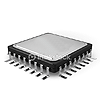#### Hardware

• Four-line × 16-character, easier-to-read LCD display
• Battery powered with solar cell assistance to lengthen battery life
• Auto Power Off
• Hard plastic, colour-coded keys
• Non-slip rubber feet
• Impact-resistant cover with quick-reference card
• Snap-on protective hard case#### Support## TI-30X Pro MultiView™ Scientific Calculator Accessories

Extend the power of your calculator with accessories for the TI-30X Pro MultiView™ scientific calculator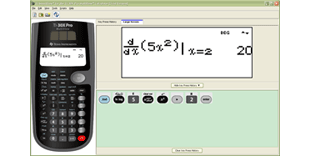## TI-SmartView™ Emulator Software

This simple software complements the TI-30X Pro MultiView™ scientific calculators, letting the teacher project a representation of the calculator’s display to the entire class. It is an ideal demonstration tool for leading classroom instruction of maths and science concepts.